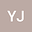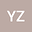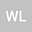Random sampling stability in weighted reproducing kernel subspaces of $L_\nu^p(\mathbb{R}^d)$
•••• Yingchun Jiang,
• Yajing Zhang,
• Wan Li
Yingchun Jiang
Guilin University of Electronic Technology

Corresponding Author:guilinjiang@126.com

Author ProfileYajing Zhang
Guilin University of Electronic Technology
Author ProfileWan Li
Guilin University of Electronic Technology
Author Profile## Abstract

In this paper, we mainly study the random sampling stability for signals in a weighted reproducing kernel subspace of $L_\nu^p(\mathbb{R}^d)$ without the additional requirement that the kernel function has symmetry. The sampling set is independently and randomly drawn from a general probability distribution over $\mathbb{R}^d$. Based on the frame characterization of weighted reproducing kernel subspaces, we first approximate the weighted reproducing kernel space by a finite dimensional subspace on any bounded domains. Then, we prove that the random sampling stability holds with high probability for all signals in weighted reproducing kernel subspaces whose energy concentrate on a cube when the sampling size is large enough.